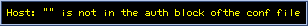Calculate 2theta (Q,d) from Lattice Constants

## CALCULATE 2theta (Q,d) FROM LATTICE CONSTANTS

First version May 22, 1999
Revised Oct. 11, 1999
Revised Aug. 2, 2000
Server changed May 6, 2001
Revised Sep. 10, 2013
K. ISHII

Some modification was done. See revised history.

Go to Old versiton### X-ray Source

Wavelength Å
Energy keV
Cu-Kα1 (1.541 Å)
Mo-Kα1 (0.7093 Å)
Ag-Kα1 (0.5594 Å)

minimum maximum

### Lattice Constants

cubic
a = Å
tetragonal
a = Å, c = Å
orthorhombic
a = Å, b = Å, c = Å
rhombohedral
a = Å, α = deg.
hexagonal
a = Å, c = Å
monoclinic
a = Å, b = Å, c = Å, β = deg.
triclinic
a = Å, b = Å, c = Å, α = deg., β = deg., γ = deg.

Standard Sample ( If you select a standard samle, symmetry is automatically determined. )
CeO2 (cubic, a = 5.411102 Å, face-center)
Si (cubic, a = 5.43102088 Å, diamond-structure)
Diamond (cubic, a = 3.56703 Å, diamond-structure)
Ge (cubic, a = 5.6575 Å, diamond-structure)
Al (cubic, a = 4.04934 Å, face-center)
Cu (cubic, a = 3.61496 Å, face-center)

### Symmetry

 Primitive Face-Center Body-Center A-Base-Center B-Base-Center C-Base-Center

### Output Type

Q ( = 4πsinθ/λ = 2π/d ) d ( = λ/2sinθ = 2π/Q )

This program is written in JaveScript, but it may not work in IE4.5 of Macintosh.

Question and Suggestion to
kenji@spring8.or.jp